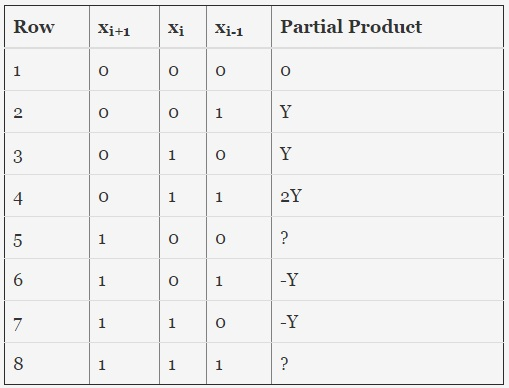# GATE | GATE IT 2006 | Question 38

• Last Updated : 29 Mar, 2018

When multiplicand Y is multiplied by multiplier X = xn – 1xn-2 ….x0 using bit-pair recoding in Booth’s algorithm, partial products are generated according to the following table.Attention reader! Don’t stop learning now.  Practice GATE exam well before the actual exam with the subject-wise and overall quizzes available in GATE Test Series Course.

Learn all GATE CS concepts with Free Live Classes on our youtube channel.

The partial products for rows 5 and 8 are
(A) 2Y and Y
(B) -2Y and 2Y
(C) -2Y and 0
(D) 0 and Y

Explanation: ALU cannot directly multiply numbers, it can only add, subtract or shift. Booth’s algorithm is a means by which we can perform multiplication with the help of addition, subtraction and shift. For performing multiplication, write both the signed numbers in binary and make the no. of bits in both equal by padding 0. Here, partial product is calculated by bit pair recoding in booth’s algorithm.

`(-2 x(i+1)+x(i)+x(i-1))Y`

So, option (C) is correct.

This solution is contributed by Shashank Shanker khare.

Quiz of this Question

My Personal Notes arrow_drop_up